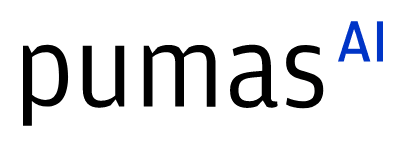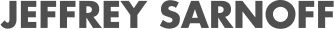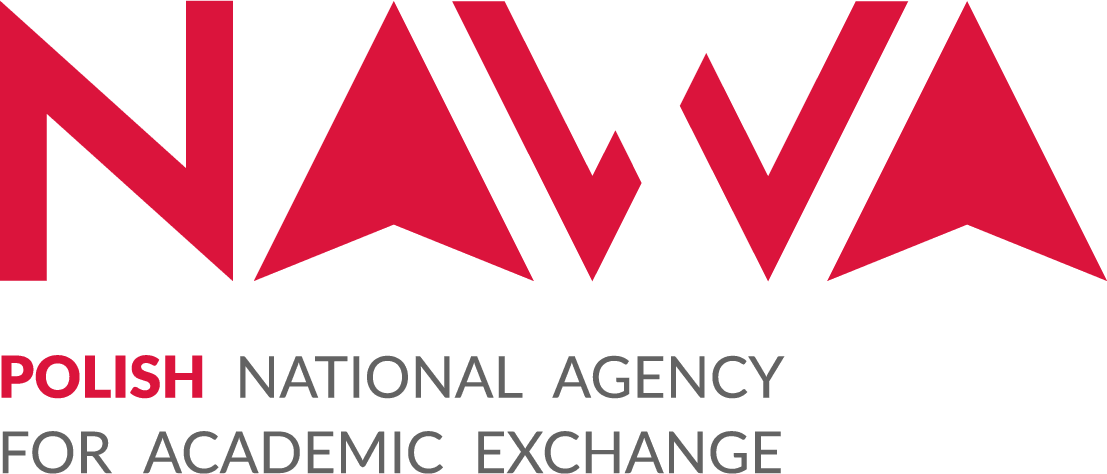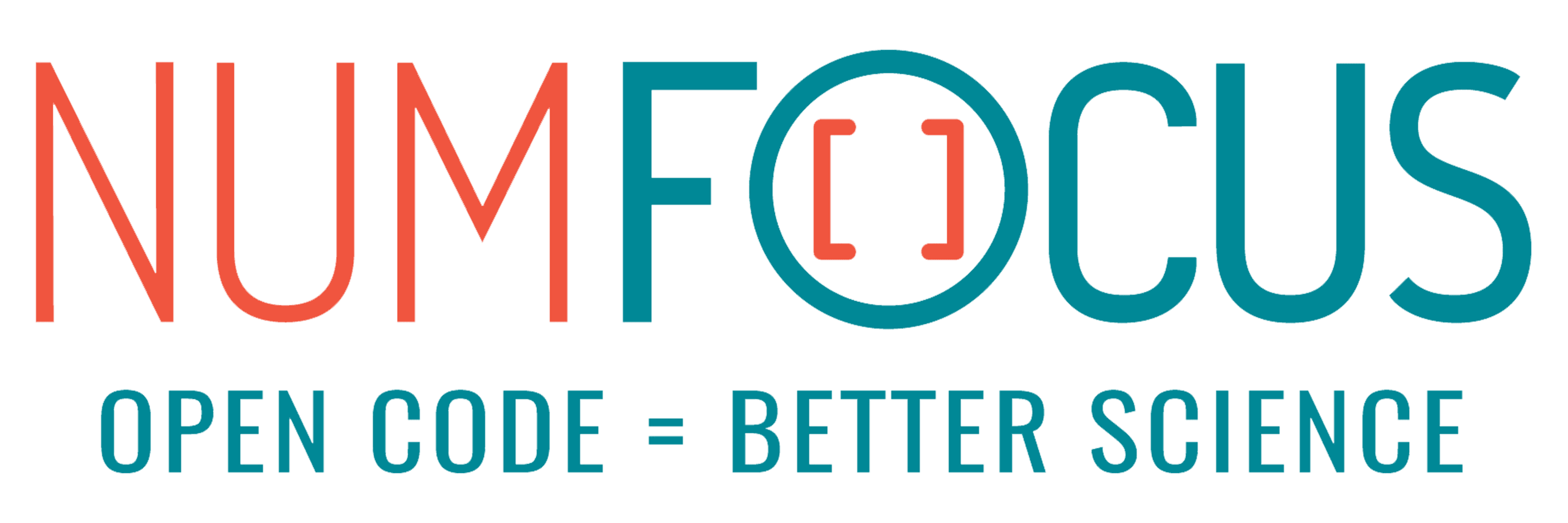# Differentiable isospectral flows for matrix diagonalization

07/28/2023, 3:30 PM3:40 PM UTC
32-D463 (Star)

#### Abstract:

In this talk, we present a differentiable Julia implementation of eigenvalue algorithms based on isospectral flows, i.e., matrix systems of ordinary differential equations (ODEs) that continuously drive Hermitian matrices toward a diagonal steady state. We discuss different options for suitable ODE solvers as well as methods for computing sensitivities, and showcase applications in quantum many-body physics.

#### Description:

One of the most important problems in numerical analysis is the design of algorithms for solving the eigenvalue problem, i.e., to find the eigenvalues and eigenvectors of a matrix. For a Hermitian matrix, this amounts to constructing a unitary transformation that brings it into a diagonal form. Traditional iterative algorithms, such as the Jacobi eigenvalue algorithm or the QR algorithm, accomplish this in a series of discrete steps. In the continuum limit, the unitary transformation is instead performed in a continuous fashion, as described by isospectral flows. Solving the ODEs modeling such an isospectral flow numerically in turn corresponds to an iterative eigenvalue algorithm. The QR algorithm, for example, emerges when sampling a particular isospectral flow at unit intervals .

Eigenvalue algorithms based on continuous isospectral flows have proven particularly useful for studying closed quantum many-body systems whose properties are determined by their Hamiltonian - a Hermitian matrix whose dimension grows exponentially with the number of system constituents. In this case, the operator structure of typical physical Hamiltonians can be exploited to introduce approximations that enable the analysis of larger systems .

Formulating eigenvalue algorithms based on continuous isospectral flows in a differentiable manner allows for the efficient and accurate computation of derivatives of physical quantities of interest, such as (time-dependent) expectation values of observables, with respect to Hamiltonian parameters. The derivative information can be utilized to perform, e.g., parameter estimation, inverse Hamiltonian design, or sensitivity analysis. For the forward simulation of the ODEs, we utilize OrdinaryDiffEq.jl and custom implementations of solvers respecting the isospectrality of the flow. To compute derivatives we utilize the automatic differentiation (AD) framework of Julia, particularly SciMLSensitivity.jl, and define custom rules for forward- and reverse-mode AD tailored toward quantum many-body problems.

 U. Helmke and J. B. Moore, Optimization and Dynamical Systems, Communications and Control Engineering Series (Springer, 1994).  S. Kehrein, The Flow Equation Approach to Many-Particle Systems, Springer Tracts in Modern Physics (Springer, 2006).